Did you know that you can use π to partition the positive integers into two disjoint groups? It's not hard. One group is generated by the integer portions of multiples of π. The FLOOR function gives the integer portion of a positive number, so you can write integer that are generated from π as Bn = {floor(n*π)} for n=1,2,3,.... This is called the Beatty sequence for π. The first few numbers in the Beatty sequence for π are 3, 6, 9, 12, 15, 18, 21, 25, 28, 31, 34, 37, 40, 43, 47, 50, 53, ....

The second group contains all the positive integers that are not in the Beatty sequence: 1, 2, 4, 5, 7, 8, 10, 11, 13, 14, 16, 17, 19, 20, 22, 23, 24, 26, .... A remarkable fact is that the second group of integers is also the Beatty sequence for some number! In fact, it is the Beatty sequence for the number π/(π-1). So, the positive integers are partitioned into two mutually disjoint groups by using the Beatty sequence for π and the complementary Beatty sequence for π/(π-1). These two sequences generate all positive integers, and no integer is in both sequences. For example, the number 2020 appears only in the Beatty sequence for π whereas 2022 appears in the complementary sequence.

It turns out that the only properties of π that are needed for this result is the fact that π is irrational and π > 1, a result that is known as Beatty's Theorem. This article uses SAS to illustrate the Beatty sequence for π and its complementary sequence.

### The Beatty sequence

For any irrational number r > 1, the Beatty sequence for r is integer portion of the sequence r, 2*r, 3*r, .... You can compute the Beatty sequence as Bn = {floor(n*r)} for n=1,2,3,.... The complementary sequence is generated by the irrational number c = r/(r-1) in the same way. The complementary sequence is Cn = {floor(n*c)} for n=1,2,3,....

With these definitions, Beatty's theorem (also called Rayleigh's theorem) states that for any irrational number, r > 1, the Beatty sequence and the complementary sequence are disjoint and generate all positive integers. For every positive integer, y, either y is an element of the Beatty sequence or y is an element of the complementary sequence. Thus, the Beatty sequence and its complementary sequence partition the integers into two disjoint parts.

For a formal proof, see the Wikipedia article or excellent "Cut the Knot" web site.

### The Beatty sequence for pi

Let's illustrate the theorem by using r = π and a finite number of positive integers. The following example uses the first 355 terms of the Beatty and complementary sequences, which cover the first 520 positive integers. Why 355 terms? Because 355/113 is part of the continued fraction expansion of π, and is an excellent approximation of π.

First, let's visualize the individual sequences. Each sequence is the restriction of a line to the postive integers. The Beatty sequence Bn = {floor(π n)} is obtained from the line through the origin with slope π; the complementary sequence Cn is obtained from the line with slope π/(π - 1) ≈ 1.4669.... The following SAS DATA step generates values for each sequence, then sorts the sequence values. The values are graphed and color-coded according to whether they belong to the Beatty sequence ('B') or the complementary sequence ('C').

```%let maxN = 355; /* because pi ~ 355/113 */ data Beatty; pi = constant('pi'); Sequence = 'B'; /* slope = pi */ do n = 1 to &maxN; s = floor(pi*n); /* the Beatty sequence */ output; end; Sequence = 'C'; /* slope = pi/(pi-1) */ do n = 1 to &maxN; s = floor(pi/(pi-1)*n); /* the complementary sequence */ output; end; /* find the lessor of the maximum values of the sequences */ MB = floor(pi* &maxN); MC = floor(pi/(pi-1)* &maxN); call symputx('maxS', min(MB, MC)); /* for maxN=355, maxS=520 */ drop MB MC pi; run;   %put &=maxS; /* display value in SAS log */ proc sort data=Beatty; by s; run;   ods graphics / width=400px height=560px; title "Beatty and Complementary Sequences for pi"; proc sgplot data=Beatty; scatter x=n y=s / group=Sequence markerattrs=(symbol=CircleFilled); xaxis grid; yaxis max=&maxS grid LABEL="Sequence Value"; run;```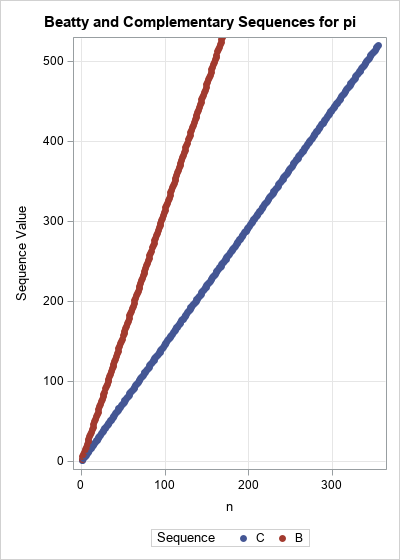The red line has slope π, and the blue line has slope π/(π-1). The domain of these functions contains the integers in [1, 355]. The range of the red line is the Beatty sequence for π; the range of the blue line is the complementary sequence.

Because of the scale, it is difficult to determine how the range of the two linear functions interlace. Let's print out a few values of the interlaced sequences:

```proc print data=Beatty(obs=12) noobs; var s Sequence; run;```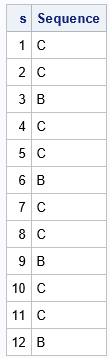The output shows that the first two integers are from the complementary sequence whereas the third is from the Beatty sequence for π. I call this the "two out, one in" pattern. This pattern continues for the first 12 observations. Now, clearly this pattern cannot continue forever, or else 1/3 of the positive integers would be in the Beatty sequence. However, we know that (1/π)th of the integers belong to the Beatty sequence by considering the limit of the ratio n/Bn as n → ∞. Thus, slightly less than 1/3 of the integers are in the Beatty sequence. Let's plot the first 60 positive integers and visualize whether each integer belongs to the Beatty sequence or the complementary sequence, as follows:

```data SeqViz; set Beatty(where=(s<=60)); z = 1; /* for plotting the points as a strip plot */ run;   ods graphics / width=640px height=150px; title "Beatty and Complementary Sequences for pi"; title2 "First 60 Integers"; proc sgplot data=SeqViz noautolegend; where s<=60; yaxis display=none; xaxis grid gridattrs=(color=CXF0F0F0) values=( 0 to 21 by 3 25 to 43 by 3 47 to 60 by 3 ) valueshint; scatter x=s y=z / group=Sequence datalabel=Sequence datalabelpos=top datalabelattrs=(size=12) markerattrs=(symbol=SquareFilled size=12); run;```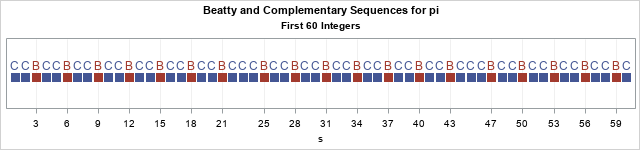The graph shows that every so often there are three (rather than two) consecutive elements of the complementary sequence. This first happens for the integers 22, 23, and 24. The fact that the "extra" element of the complementary sequence appears at 22 is not random. It is related to the fact that 22/7 is a good approximation to π. After that, the pattern returns to "two out, one in" until the next multiple of 22, which is 44, when another triplet of integers belong to the complementary sequence.

The pattern deviates again for other numerators that are associated with the continued fraction expansion of π, such as 355/113. The next graph shows the membership of integers in the range [302, 361]. The pattern of "two out, one in" is broken at 308 = 22*14 and at 330=22*15, but then the pattern deviates again at 355, which is not a multiple of 22.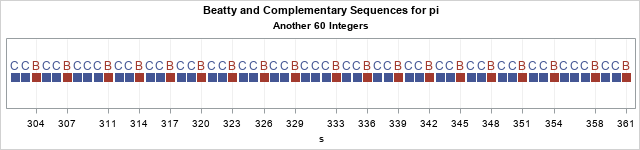### Check that the Beatty and complementary sequences are disjoint

We have asserted that the Beatty and complementary sequences are disjoint and that their union is the set of all positive integers. You can't use numerical computations to prove this fact, but you can verify that it is true for the finite set of numbers that we have generated. The following SAS/IML program checks that the two sequences do not intersect and that their union covers all positive integers (up to 520).

```/* Check union and intersection properties of Beatty and complementary sequences */ proc iml; use Beatty; read all var 's' into B where(Sequence='B'); read all var 's' into C where(Sequence='C'); close;   /* is there any intersection? */ intersect = xsect(B, C); if IsEmpty(intersect) then print "B and C do not intersect"; else print "The intersection is:", intersect;   /* use the UNION function */ max = min( max(B), max(C) ); union = union(B, C); union = union[ , 1:max]; /* only test for values in [1, max] */ if all(union = 1:max) then print "All integers are found"; else print "Some integer is missing"; QUIT;```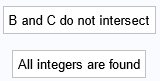The program verifies (for positive integers less than 520) that the Beatty and complementary sequences do not have any intersection. Furthermore, each integer is either an element of the Beatty sequence for π or it is an element of the complementary sequence.

I want to point out a cool SAS/IML syntax: The IF-THEN statement can be used to test whether one vector equals another vector. In the program, the statement all(union = 1:max) returns true is every element of the vector union is equal to the corresponding element of the vector {1,2,3,...,max}.

### Summary

This article describes Beatty's theorem, which is an interesting result in mathematics. Given any irrational number, r > 1, the Beatty sequence for r and the complementary sequence partition the positive integers into two disjoint groups: the Beatty sequence for r and the complementary sequence. The article illustrates the theorem by using r = π and shows some connections with the continued fraction expansion of π.

The post The Beatty sequence for pi appeared first on The DO Loop.